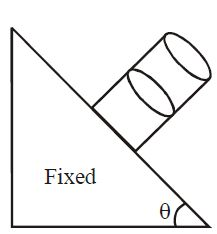Angle made by water surface!A cylindrical vessel filled with water is released on an inclined surface of angle $\theta$ as shown in figure. The friction coefficient of surface with vessel is ($\mu < \tan \theta$). Then the constant angle made by the surface of water with the incline will be:

×